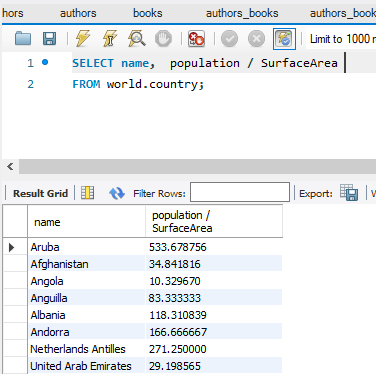1.4

# Arithmetic Operators

### Arithmetic Operators

• Arithmetic operators can be used in the SELECT, WHERE, and ORDER BY clauses.
• Operators are evaluated in the same way as arithmetic in other contexts.

Table 4. Operators and precedence order

Operator Name Order of Precedence

*

Multiplication

1

/

Division

1

DIV

Integer Division

1

% (MOD)

Modulo (remainder)

1

+

2

-

Subtraction

2

Code Example:

USE world;SELECT name, population / SurfaceAreaAS "People per square mile"FROM country;

Results: